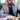# Binarysearch.io Collecting Coins Solution

January 03, 2021

### Problem statement

You are given a two-dimensional integer `matrix` where each cell represents number of coins in that cell. Assuming we start at `matrix`, and can only move right or down, find the maximum number of coins you can collect by the bottom right corner.

### Intuition

The key idea is that every step i.e. `(i, j)` we have a choice wether to go right, i.e. `(i+1, j)` or to go bottom `(i, j+1)`. We use that to come up with a recursive formulation:

`optimal_path(i, j) = matrix[i][j] + max(optimal_path(i+1, j), optimal_path(i, j+1))`

Following is the naive recursive code:

#### Recursive code

``````    def solve(self, matrix):
M, N = len(matrix), len(matrix)
def optimal_path(i, j):
if i >= M or j >= N: return 0
return matrix[i][j] + max(optimal_path(i + 1, j), optimal_path(i, j + 1))
return optimal_path(0, 0)``````

### Implementation

#### Memoized code

``````    def solve(self, matrix):
M, N = len(matrix), len(matrix)
memo = {}
def optimal_path(i, j):
if (i, j) in memo:
return memo[(i, j)]
if i >= M or j >= N:
return 0
output = matrix[i][j] + max(optimal_path(matrix, i + 1, j), optimal_path(matrix, i, j + 1))
memo[(i, j)] = output
return output
return optimal_path(0, 0)``````

#### Top-Down Dynamic Programming

``````    def solve(self, matrix):
M, N = len(matrix), len(matrix)
dp = [*(N+1) for i in range(M+1)]
for i in range(1, M+1):
for j in range(1, N+1):
dp[i][j] = matrix[i-1][j-1] + max(dp[i-1][j], dp[i][j-1])
return dp[M][N]``````

### Time complexity

O(N**2) for the memoized and dynamic programming solutions

### Space complexity

O(N**2)Written by Ganesh Iyer A software engineer building platforms for leveraging artificial intelligence in healthcare. Follow me on twitter